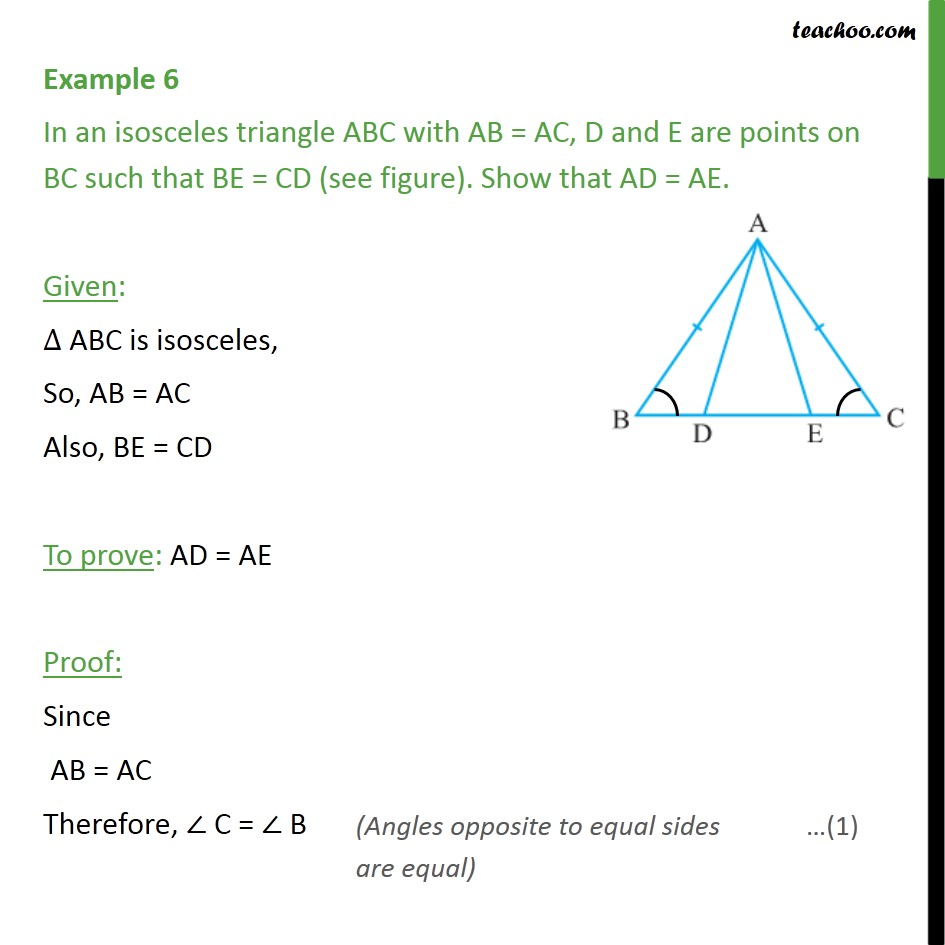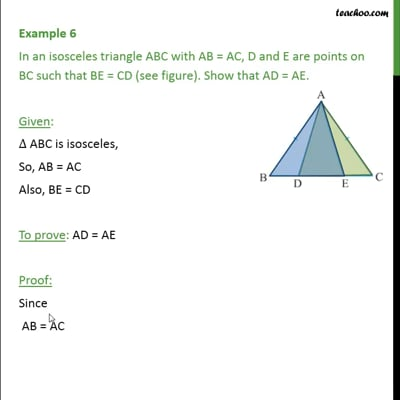Examples

Chapter 7 Class 9 Triangles
Serial order wiseThis video is only available for Teachoo black users

Maths Crash Course - Live lectures + all videos + Real time Doubt solving!

### Transcript

Example 6 In an isosceles triangle ABC with AB = AC, D and E are points on BC such that BE = CD (see figure). Show that AD = AE. Given: ∆ ABC is isosceles, So, AB = AC Also, BE = CD To prove: AD = AE Proof: Since AB = AC Therefore, ∠ C = ∠ B In ∆ ACD and ∆ ABE, AC = AB ∠ C = ∠ B CD = BE So, ∆ ACD ≅ ∆ ABE ∴ AD = AE Hence proved